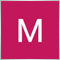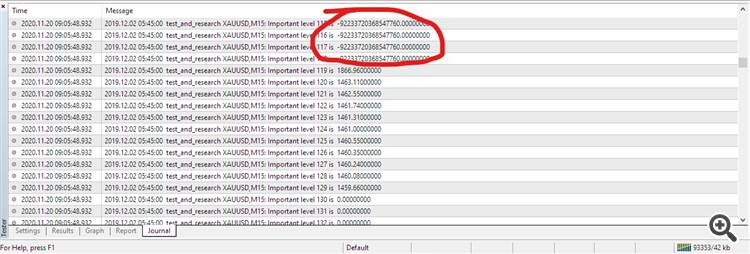# Algorithm to detect support and resistance - page 290

Hi guys, this is how I define support and resistance to be coded.

First determine how many bars we want to use... for example its 500...

Then determine the range between highest and lowest in that bars ( for example 500 pips ).

Next, we let the user input the length of the legs of the support or resistance level in percentage based on the full range ( 500 pips that we talked before ).

So if the user input 30 %, then the two legs of support and resistance must at least have 150 pips.

So anyone has solution how to code this ?

In pseucode frist...Moderator
31752

Comments that do not relate to this topic, have been moved to "Off Topic Posts".113112

Hello guys,

• I tried @difenp Algorithm for the S/R Level.
• Used the same Logic.
• Stored the Output into an array.
• Sorted that Srray and printed to see the outputs.
• Result : Strangely, The results gave many negative results.
• Question: How is it possible for this algorithm fto chuck out Negative value when the price has never been negative?
• Can somebody explain this?

I have attached thge source code as separate file. Screeshot of the terminal is alsO includedCode for your direct check here:

```

#property strict

#property  show_inputs
extern int loopback=800, period = 60;

//+------------------------------------------------------------------+

//| Expert initialization function                                   |

//+------------------------------------------------------------------+

int OnInit()

{

int commonPoint;

int minLevel, maxLevel;

double highest, lowest, stepIncrement, tolerance, high;

double storeLevel;

///ArrayResize(storeLevel, loopback);

minLevel = iLowest(Symbol(), Period(), MODE_LOW, loopback, 0);

maxLevel = iHighest(Symbol(), Period(), MODE_HIGH, loopback, 0);

highest = iHigh(Symbol(), Period(), maxLevel);

lowest = iLow(Symbol(), Period(), minLevel);

printf("Max Level = " +highest + "Min Level = " +lowest);

//Print("max: " + highest + " min: " + lowest);

stepIncrement = 200*Point;

tolerance = 100*Point;

static double tmp;

tmp = 0.0;

for(double actPrice = lowest; actPrice <= highest; actPrice += stepIncrement)

{

for(int i = 1; i <= loopback; i++)

{

//do some stuff here...

high = iHigh(Symbol(), Period(), i);

double topRange, bottomRange;

/**  if is the first value tmp stores the first high encountered until that moment **/

if(tmp == 0)

{

tmp = high;

}

else

{

//define a buffer adding a subtracting from tmp to check if the new high is within that value

topRange = tmp + tolerance;

bottomRange = tmp - tolerance;

if(high <= topRange && high >= bottomRange)

{

commonPoint++;

}

}

//if has been touched at least three times reset common point

//tmp goes to the new high value to keep looping

if(commonPoint == 3)

{

commonPoint = 0;

tmp = high;

///Print("valore tmp: " + tmp);

storeLevel[i] = tmp;

}

}

}

ArraySort(storeLevel,WHOLE_ARRAY,0,MODE_DESCEND);// sorting the array to print

for(int i= loopback; i>0; i--)// loop for printing

{

printf("Important level " +i +" is  " + DoubleToStr(NormalizeDouble(storeLevel[i],2)));

}

return(INIT_SUCCEEDED);

}

//+------------------------------------------------------------------+

//| Expert deinitialization function                                 |

//+------------------------------------------------------------------+

void OnDeinit(const int reason)

{

//---

}

//+------------------------------------------------------------------+

//| Expert tick function                                             |

//+------------------------------------------------------------------+

void OnTick()

{

}

```
Files: